# The Bellman Equation

## Introduction

Those who have come across reinforcement learning(RL) are well aware that the Bellman Equation is an unavoidable part of RL whose application can be found throughout RL in one form or the other. The Bellman equation helps generate more calculated and better-bearing results by combining different RL functions.

Before we dive deep into the Bellman equation, let’s learn some get back to basics for understanding its application in a better way.

## Understanding The Essential Keywords

#### Agent(A)

The term agent is referred to the entity that performs the actions in a given environment.

#### Environment()

The environment can be defined as a situation under observation in which our agent is working.

#### State(S)

The state can be defined as the result after at the end of every action taken before the reward.

#### Reward(R)

A reward is feedback gained after the end of the complete cycle of the agent.

#### Policy(π)

The policy represented by π is used to define the motive behind the action taken by the agent in the cycle. Mathematically policy can be stated as the set of actions in a given state, giving the probability of the following action the agent will choose.

## Markov’s Decision Process(MDP)

As the name suggests, Markov’s decision process can be defined as the property of cumulative actions that lie in accordance with the Markov property which states that the decision taken in the given environment is the result of a positive signal generated by the system. Markov's Decision process in RL is used for decision-making with respect to long-term rewards in the current state.

In the above image, as we can see, there are a few alphabets assigned to the processes. Let’s define and understand them.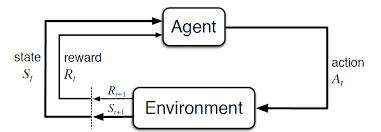• S: It is the set of all possible states in a process.
• A: It is the set of all possible actions the agent can take.
• R: It is the set of all possible rewards the agent can acquire at the end of a cycle
• Rt+1: It is the reward obtained at a given time t.
• St+1: Is the state obtained at a given time t.

Let’s understand how this works altogether.

Our decision-maker or the agent takes action in the current state, looking at the situation that moves our environment to a different state, generating rewards as the outcome. Finite MDP following Markov’s property works only in the current state and takes decisions based on the current scenario and does not depend on past actions or rewards. The agent works in the present state, working in an optimized manner for future rewards.

Two more additions to Markov's process named as the reward function R and the discount factor γ are the following additions to our equation of the MDP.

The reward function signifies the total number of rewards attained at the end of the cycle, and Gamma shows the discount rate where 0<γ≤1.

Gama is used to make the future less uncertain; thus, the gamma is used to limit the contribution of the future rewards in our cycle.

The Markov reward process(MRP) in terms of RL evaluates the most optimized pathway to travel through the basis of maximum rewards attained. The formulae we use for the reward are as shown below.

As we can see in the image above, each path or state that the agent takes has either positive or negative rewards based on what the agent does after waking up. The same is used to calculate the reward of the whole cycle, and the most optimized path is computed using the above-stated MDP formulae.

In reinforcement learning we use value functions along with MDP to categorize the best action that one agent should perform in a given environment to attain maximum reward. Let's have a look at how these value functions look and later in this article we would be studying in depth about them.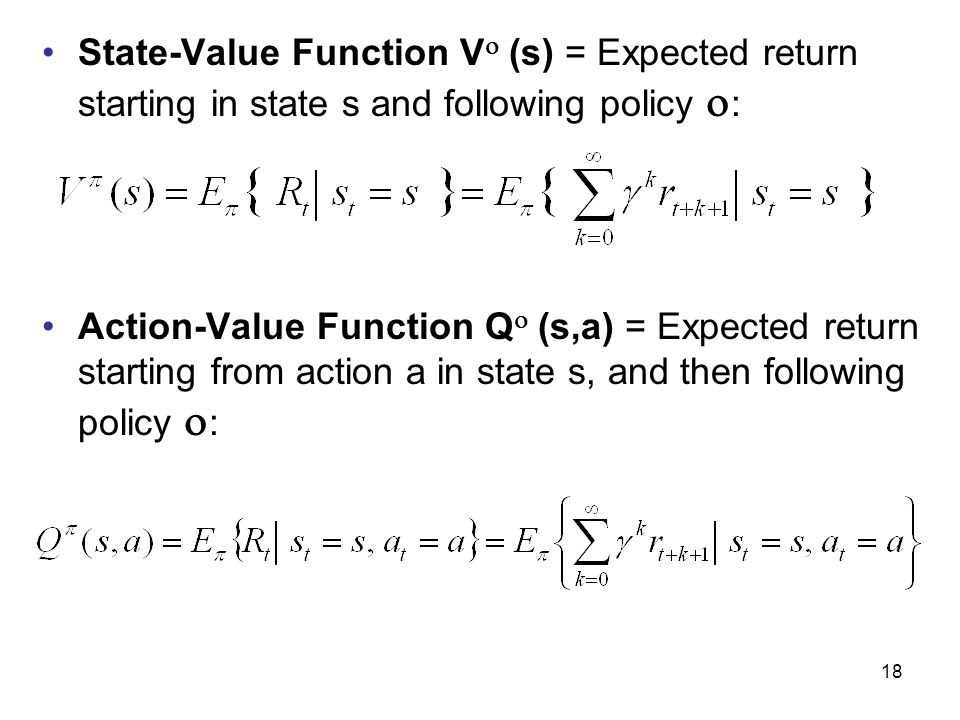Now that we are aware of what state-value function and action-value function look like and how they help the agent predict and find the most optimized beneficial move that the agent can make to attain better reward, let's learn in depth about them.

## State-Value Function

State-Value function talks about the function of the state that deals with the long-term value of the state i.e., it helps predict the long-term reward from the current that would be obtained from the whole cycle by the agent. Let’s understand this with the help of a formula.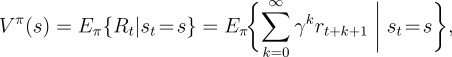Where Vπ (s)  represents the value function under a state

Eπ represents the expected state-value in the given time t.

In the case of the state value function, the function Vπ (s) defines how suitable the state is to be for the agent at the given time t with current state s following the policy π.

## Action-Value Function

The action-value function returns the value of the action taken in the current state that follows the policy π. It signifies how beneficial it would be for the agent to take the probable action in the current state, which gives the most beneficial return and better rewards.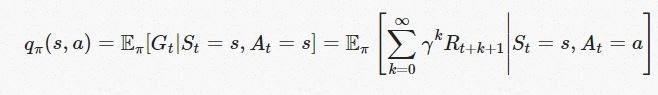Where qπ (s, a) represents the action and the state

Eπ represents the stochasticity in the environment

The formulae are used to calculate the probable reward that the agent will get on taking action in the state that helps us determine the quality of the action.

## Optimal Policy

The optimal policy talks about the maximum number of rewards that the agent can secure by taking the right actions in the current state. Algorithms in RL focuses on implementing optimal policy to the environments that follow it.

The goal of the optimal policy is to calculate the policy that would generate the most optimal rewards compared to other probable, possible policies.

## Bellman Equation

The Bellman equation helps provide a standard representation of all the value functions mentioned above and helps them break the problem into two simpler parts that are: immediate reward and the discounted future values corresponding to the action taken by the agent in the current state.

Below you will see the Bellman equation with all the constraints defined.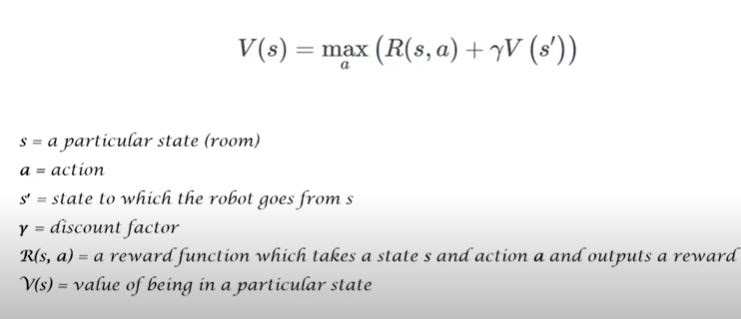### Bellman Equation For State-Value Function

Since we have already defined the state-value function before and are well aware of its functioning now, let’s understand how it works with the bellman equation.

In the case of the state-value function, the bellman equation helps choose the most optimized state that followed the policy in the given environment recursively. Let’s understand it with the given formulae and backup diagram.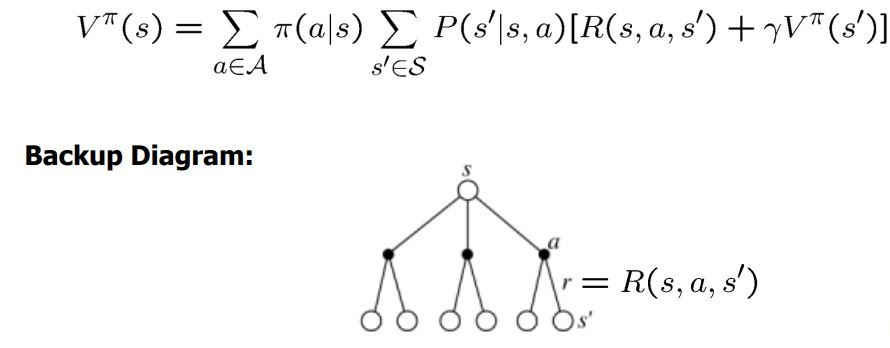Here in the formulae, we can see that the Bellman equation breaks the state into two parts: the P(s`|s, a) the expected reward of the taken state and the successor with the discount rate.

### Bellman Equation For Action-Value Function

Similar to the working of the state-value function, the Bellman equation for the action-value function helps us calculate the state-action air, i.e., the state the agent is currently in, along with the expected action the agent would take in the environment.

In the formulae given below, since we are calculating both action and state pair, we take the sum of both, i.e., Q(s, a) that follows the policy π.

The Backup diagrams help explain the working of the active-value function for the bellman equations.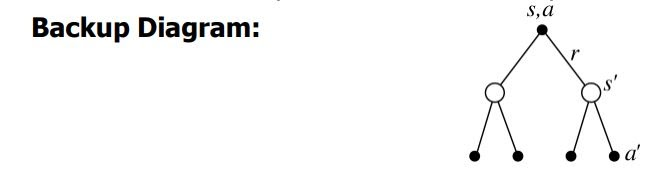## Bellman Optimality Equations

The role of the bellman optimality equation is to find the most optimal policy that the agent can follow to get the best cumulative reward when the cycle ends. Since we have already seen the state-value and active-value function in the above state, let’s move on to the optimal equation looking at the same.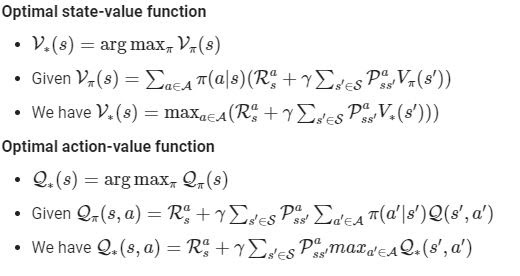This equation can be solved without the use of MDP simply by applying Bellman Equation, but usually, this doesn’t happen in our day-to-day stated problems as the value of R(s, a) and Pass` is not known.

The backup diagrams below provide us with a graphical representation of the future states and actions that our agent can achieve at the end of our cycle.

Note* The diagram on the left is for the active-value function, and that on the right is for the state-value function.

The Bellman optimality equation states, conceptually, that the reward of any state within an optimal policy has to be the return the agent receives whenever it executes the optimal policy's best action.

Q1. What are the real-world examples of the application of the Bellman equation?

Ans: The best examples can be seen in the finance sector, where the machine makes calculative steps to maximize the total rewards gained in a cycle. In human cognitive behavior also we can see a similar pattern where ideally humans work towards achieving the suitable reward.

Q2. Why is discount used so extensively in MDP and Bellman Equation?

Ans: The simple answer is to have finite mathematical solutions MDP for the given cycle in an environment, reducing the uncertainty of the future.

Q3.What is the Bellman Error?

Ans: The Bellman error is the discrepancy between the Q(s, a) estimate and the expected next reward and state-action value.

## Key Takeaways

In this article, we have briefly discussed the mathematical application of the Bellman Equation in reinforcement learning. Bellman equation helps generate better long-term rewards in a given environment by assisting the agent in making the right move in the current state to gain better rewards in the future state.

We have also covered Markov’s Decision Process and various forms of the Bellman equation in active-value function, state value function, and the optimization policy individually and in terms of Bellman Equation in Reinforcement Learning. o learn more about reinforcement learning follow our blogs to have a better understanding of the subject.